## Maximum ROP Before Fracture Formation

In this topic, we will apply the effective mud density formula to determine maximum ROP before fracturing formation.Figure 1 – Max ROP before Fracturing Formation

These two equations that will be used to determine the maximum ROP are listed below;

Effective mud density due to cuttings in the hole can be determined by the empirical equation below;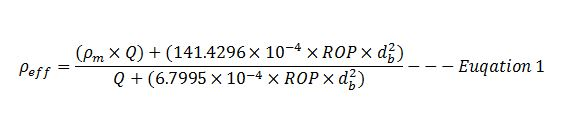Where;

ρeff is effective mud density in ppg.

ρm is mud density in ppg.

Q is flow rate in gpm.

ROP is rate of penetration in fph.

db is wellbore diameter or bit diameter in inch.

## Increase In Mud Weight Due To Cutting

Cutting generated while drilling will increase drilling fluid density and it will finally affect equivalent circulating density while drilling. In this topic, we will talk about how to determine mud weight increase due to cutting.Figure 1 – Cutting Increases Mud Density

Effective mud density due to cuttings in the hole can be determined by the empirical equation below;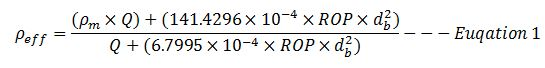## Equivalent Circulating Density (ECD) Using Yield Point for MW More than 13 ppg

Another equation to determine equivalent circulating density is to use yield point.This formula below is used to calculate the ECD and it is good for mud weight more than to 13.0 ppg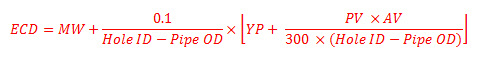Where:

ECD is equivalent circulating density in ppg.

MW is mud weight in ppg.

Hold ID is inside diameter of hole in inch.

Pipe OD is outside diameter of pipe in inch.

YP is mud yield point.

YP can be calculated by these following equations.

YP = Reading at 300 rpm – PV

PV = Reading at 600 rpm – Reading at 300 rpm

AV is annular velocity in ft/min

AV can be determined by the following equation.

AV in ft/min = (24.5 x Q) ÷ (Dh2 – Dp2)

where

Q = flow rate in gpm

Dh = inside diameter of casing or hole size in inch

Dp = outside diameter of drill pipe, drill collars, or tubing in inch

## Equivalent Circulating Density (ECD) Using Yield Point for MW less than 13 ppg

Another way to determine equivalent circulating density (ECD) is to use yield point.This formula below is used to calculate the ECD and it is good for mud weight less than or equal to 13.0 ppg.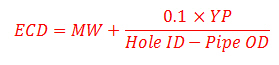Where:

ECD is equivalent circulating density in ppg

MW is mud weight in ppg

Hold ID is inside diameter of hole in inch

Pipe OD is outside diameter of pipe in inch

YP is mud yield point

YP can be calculated by these following equations

YP = Reading at 300 rpm – PV

PV = Reading at 600 rpm – Reading at 300 rpm

## Effect of Frictional Pressure on ECD while Forward CirculationIn this article, we will describe the effect of friction pressure on bottom hole pressure and equivalent circulating density while performing forward circulation.

### What is forward circulation?

It is the typical circulating path which is from a mud pump into drill pipe. Mud is pumped down into drill string / BHA and come out of a bit.  Then, the mud is flown up the annulus and return back to surface as you can see in the diagram below (Figure 1).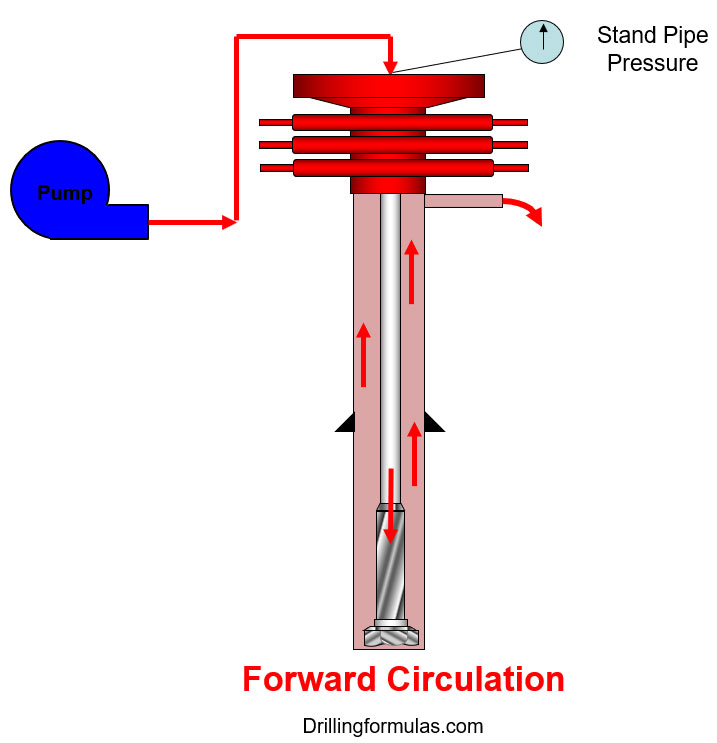Figure 1 – Forward Circulation Path